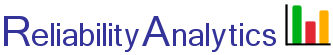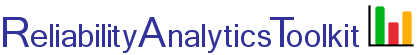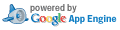# Binomial Probability of Success

Given a random sample of n items and a probability of failure/defect rate p, this tool calculates the probability that exactly x failures will occur in the sample. The probability that exactly x failures will occur in a random sample of n items is given by: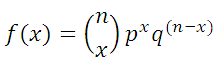The tool also calculates the cumulative probability that not more than r failures will occur in a sample of n items. The cumulative probability that r or fewer failures will occur in a sample of n items is given by: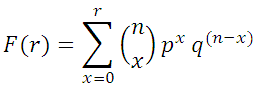where q = 1 - p.

For example, a manufacturing process creates defects at a rate of 2.5% (p=0.025). A sample of 20 parts is randomly selected (n=20). What is the probability that the sample contains 3 or fewer defective parts (r=3)? The probability of finding 3 or fewer defects in 20 samples is 0.9986.

## Calculation Inputs:

1. Number of samples (n):
2. Probability of failure/defect rate (p):
3. Maximum allowable defects (r):
4. Decimal places:
5. Chart options:

f(x) chart
F(r) chart

Title:

## Featured Reference: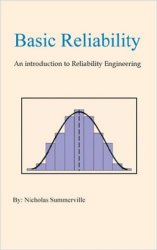Basic Reliability: An introduction to Reliability Engineering

Toolkit Home